# bayeslm

Create Bayesian linear regression model object

## Syntax

PriorMdl = bayeslm(NumPredictors)
PriorMdl = bayeslm(NumPredictors,'ModelType',modelType)
PriorMdl = bayeslm(NumPredictors,'ModelType',modelType,Name,Value)

## Description

To create a Bayesian vector autoregression (VARX) model for multivariate time series analysis, see bayesvarm.

example

PriorMdl = bayeslm(NumPredictors) creates a Bayesian linear regression model object (PriorMdl) composed of NumPredictors predictors, an intercept, and a diffuse, joint prior distribution for β and σ2. PriorMdl is a template that defines the prior distributions and dimensionality of β.

example

PriorMdl = bayeslm(NumPredictors,'ModelType',modelType) specifies the joint prior distribution modelType for β and σ2. For this syntax, modelType can be: 'conjugate', 'semiconjugate', or 'diffuse' to create a standard Bayesian linear regression prior model'mixconjugate', 'mixsemiconjugate', or 'lasso' to create a Bayesian linear regression prior model for predictor variable selection For example, 'ModelType','conjugate' specifies conjugate priors for the Gaussian likelihood, that is, β|σ2 as Gaussian, σ2 as inverse gamma.

example

PriorMdl = bayeslm(NumPredictors,'ModelType',modelType,Name,Value) uses additional options specified by one or more name-value pair arguments. For example, you can specify whether to include a regression intercept or specify additional options for the joint prior distribution modelType. If you specify 'ModelType','empirical', you must also specify the BetaDraws and Sigma2Draws name-value pair arguments. BetaDraws and Sigma2Draws characterize the respective prior distributions.If you specify 'ModelType','custom', you must also specify the LogPDF name-value pair argument. LogPDF completely characterizes the joint prior distribution. 

## Examples

collapse all

Consider the multiple linear regression model that predicts the US real gross national product (GNPR) using a linear combination of industrial production index (IPI), total employment (E), and real wages (WR).

${\text{GNPR}}_{t}={\beta }_{0}+{\beta }_{1}{\text{IPI}}_{t}+{\beta }_{2}{\text{E}}_{t}+{\beta }_{3}{\text{WR}}_{t}+{\epsilon }_{t}.$

For all $t$, ${\epsilon }_{t}$ is a series of independent Gaussian disturbances with a mean of 0 and variance ${\sigma }^{2}$.

Suppose that the regression coefficients $\beta =\left[{\beta }_{0},...,{\beta }_{3}{\right]}^{\prime }$ and the disturbance variance ${\sigma }^{2}$ are random variables, and their prior values and distribution are unknown. In this case, use the noninformative Jefferys prior: the joint prior distribution is proportional to $1/{\sigma }^{2}$.

These assumptions and the data likelihood imply an analytically tractable posterior distribution.

Create a diffuse prior model for the linear regression parameters, which is the default model type. Specify the number of predictors p.

p = 3; Mdl = bayeslm(p)
Mdl = diffuseblm with properties: NumPredictors: 3 Intercept: 1 VarNames: {4x1 cell} | Mean Std CI95 Positive Distribution ----------------------------------------------------------------------------- Intercept | 0 Inf [ NaN, NaN] 0.500 Proportional to one Beta(1) | 0 Inf [ NaN, NaN] 0.500 Proportional to one Beta(2) | 0 Inf [ NaN, NaN] 0.500 Proportional to one Beta(3) | 0 Inf [ NaN, NaN] 0.500 Proportional to one Sigma2 | Inf Inf [ NaN, NaN] 1.000 Proportional to 1/Sigma2 

Mdl is a diffuseblm Bayesian linear regression model object representing the prior distribution of the regression coefficients and disturbance variance. bayeslm displays a summary of the prior distributions at the command line. Because the prior is noninformative and the model does not contain data, the summary is trivial.

If you have data, then you can estimate characteristics of the posterior distribution by passing the prior model Mdl and data to estimate.

Consider the linear regression model in Default Diffuse Prior Model. Assume these prior distributions:

• $\beta |{\sigma }^{2}\sim {N}_{4}\left(M,V\right)$. $M$ is a 4-by-1 vector of means, and $V$ is a scaled 4-by-4 positive definite covariance matrix.

• ${\sigma }^{2}\sim IG\left(A,B\right)$. $A$ and $B$ are the shape and scale, respectively, of an inverse gamma distribution.

These assumptions and the data likelihood imply a normal-inverse-gamma semiconjugate model. The conditional posteriors are conjugate to the prior with respect to the data likelihood, but the marginal posterior is analytically intractable.

Create a normal-inverse-gamma semiconjugate prior model for the linear regression parameters. Specify the number of predictors p.

p = 3; Mdl = bayeslm(p,'ModelType','semiconjugate') 
Mdl = semiconjugateblm with properties: NumPredictors: 3 Intercept: 1 VarNames: {4x1 cell} Mu: [4x1 double] V: [4x4 double] A: 3 B: 1 | Mean Std CI95 Positive Distribution ------------------------------------------------------------------------------- Intercept | 0 100 [-195.996, 195.996] 0.500 N (0.00, 100.00^2) Beta(1) | 0 100 [-195.996, 195.996] 0.500 N (0.00, 100.00^2) Beta(2) | 0 100 [-195.996, 195.996] 0.500 N (0.00, 100.00^2) Beta(3) | 0 100 [-195.996, 195.996] 0.500 N (0.00, 100.00^2) Sigma2 | 0.5000 0.5000 [ 0.138, 1.616] 1.000 IG(3.00, 1) 

Mdl is a semiconjugateblm Bayesian linear regression model object representing the prior distribution of the regression coefficients and disturbance variance. bayeslm displays a summary of the prior distributions at the command line. For example, the elements of Positive represent the prior probability that the corresponding parameter is positive.

If you have data, then you can estimate characteristics of the marginal or conditional posterior distribution by passing the prior model Mdl and data to estimate.

Consider the linear regression model in Default Diffuse Prior Model. Assume these prior distributions:

• $\beta |{\sigma }^{2}\sim {N}_{4}\left(M,{\sigma }^{2}V\right)$. $M$ is a 4-by-1 vector of means, and $V$ is a scaled 4-by-4 positive definite covariance matrix. Suppose you have prior knowledge that $M={\left[\begin{array}{cccc}-20& 4& 0.1& 2\end{array}\right]}^{\prime }$ and V is the identity matrix.

• ${\sigma }^{2}\sim IG\left(A,B\right)$. $A$ and $B$ are the shape and scale, respectively, of an inverse gamma distribution.

These assumptions and the data likelihood imply a normal-inverse-gamma conjugate model.

Create a normal-inverse-gamma conjugate prior model for the linear regression parameters. Specify the number of predictors p and set the regression coefficient names to the corresponding variable names.

p = 3; Mdl = bayeslm(p,'ModelType','conjugate','Mu',[-20; 4; 0.1; 2],'V',eye(4),... 'VarNames',["IPI" "E" "WR"])
Mdl = conjugateblm with properties: NumPredictors: 3 Intercept: 1 VarNames: {4x1 cell} Mu: [4x1 double] V: [4x4 double] A: 3 B: 1 | Mean Std CI95 Positive Distribution ---------------------------------------------------------------------------------- Intercept | -20 0.7071 [-21.413, -18.587] 0.000 t (-20.00, 0.58^2, 6) IPI | 4 0.7071 [ 2.587, 5.413] 1.000 t (4.00, 0.58^2, 6) E | 0.1000 0.7071 [-1.313, 1.513] 0.566 t (0.10, 0.58^2, 6) WR | 2 0.7071 [ 0.587, 3.413] 0.993 t (2.00, 0.58^2, 6) Sigma2 | 0.5000 0.5000 [ 0.138, 1.616] 1.000 IG(3.00, 1) 

Mdl is a conjugateblm Bayesian linear regression model object representing the prior distribution of the regression coefficients and disturbance variance. bayeslm displays a summary of the prior distributions at the command line. Although bayeslm assigns names to the intercept and disturbance variance, all other coefficients have the specified names.

By default, bayeslm sets the shape and scale to 3 and 1, respectively. Suppose you have prior knowledge that the shape and scale are 5 and 2.

Set the prior shape and scale of ${\sigma }^{2}$ to their assumed values.

Mdl.A = 5; Mdl.B = 2
Mdl = conjugateblm with properties: NumPredictors: 3 Intercept: 1 VarNames: {4x1 cell} Mu: [4x1 double] V: [4x4 double] A: 5 B: 2 | Mean Std CI95 Positive Distribution ---------------------------------------------------------------------------------- Intercept | -20 0.3536 [-20.705, -19.295] 0.000 t (-20.00, 0.32^2, 10) IPI | 4 0.3536 [ 3.295, 4.705] 1.000 t (4.00, 0.32^2, 10) E | 0.1000 0.3536 [-0.605, 0.805] 0.621 t (0.10, 0.32^2, 10) WR | 2 0.3536 [ 1.295, 2.705] 1.000 t (2.00, 0.32^2, 10) Sigma2 | 0.1250 0.0722 [ 0.049, 0.308] 1.000 IG(5.00, 2) 

bayeslm updates the prior distribution summary based on the changes in the shape and scale.

Consider the linear regression model in Default Diffuse Prior Model. Assume these prior distributions:

•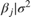is 4-D t distribution with 50 degrees of freedom for each component and the identity matrix for the correlation matrix. Also, the distribution is centered at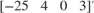and each component is scaled by the corresponding elements of the vector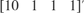.

•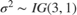.

bayeslm treats these assumptions and the data likelihood as if the corresponding posterior is analytically intractable.

Declare a MATLAB® function that:

• Accepts values of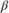and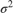together in a column vector, and accepts values of the hyperparameters

• Returns the value of the joint prior distribution,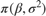, given the values ofandfunction logPDF = priorMVTIG(params,ct,st,dof,C,a,b) %priorMVTIG Log density of multivariate t times inverse gamma % priorMVTIG passes params(1:end-1) to the multivariate t density % function with dof degrees of freedom for each component and positive % definite correlation matrix C. priorMVTIG returns the log of the product of % the two evaluated densities. % % params: Parameter values at which the densities are evaluated, an % m-by-1 numeric vector. % % ct: Multivariate t distribution component centers, an (m-1)-by-1 % numeric vector. Elements correspond to the first m-1 elements % of params. % % st: Multivariate t distribution component scales, an (m-1)-by-1 % numeric (m-1)-by-1 numeric vector. Elements correspond to the % first m-1 elements of params. % % dof: Degrees of freedom for the multivariate t distribution, a % numeric scalar or (m-1)-by-1 numeric vector. priorMVTIG expands % scalars such that dof = dof*ones(m-1,1). Elements of dof % correspond to the elements of params(1:end-1). % % C: Correlation matrix for the multivariate t distribution, an % (m-1)-by-(m-1) symmetric, positive definite matrix. Rows and % columns correspond to the elements of params(1:end-1). % % a: Inverse gamma shape parameter, a positive numeric scalar. % % b: Inverse gamma scale parameter, a positive scalar. % beta = params(1:(end-1)); sigma2 = params(end); tVal = (beta - ct)./st; mvtDensity = mvtpdf(tVal,C,dof); igDensity = sigma2^(-a-1)*exp(-1/(sigma2*b))/(gamma(a)*b^a); logPDF = log(mvtDensity*igDensity); end 

Create an anonymous function that operates like priorMVTIG, but accepts the parameter values only and holds the hyperparameter values fixed.

dof = 50; C = eye(4); ct = [-25; 4; 0; 3]; st = [10; 1; 1; 1]; a = 3; b = 1; prior = @(params)priorMVTIG(params,ct,st,dof,C,a,b); 

Create a custom joint prior model for the linear regression parameters. Specify the number of predictors p. Also, specify the function handle for priorMVTIG, and pass the hyperparameter values.

p = 3; Mdl = bayeslm(p,'ModelType','custom','LogPDF',prior) 
Mdl = customblm with properties: NumPredictors: 3 Intercept: 1 VarNames: {4x1 cell} LogPDF: @(params)priorMVTIG(params,ct,st,dof,C,a,b) The priors are defined by the function: @(params)priorMVTIG(params,ct,st,dof,C,a,b) 

Mdl is a customblm Bayesian linear regression model object representing the prior distribution of the regression coefficients and disturbance variance. In this case, bayeslm does not display a summary of the prior distributions at the command line.

Consider the linear regression model in Default Diffuse Prior Model.

Assume these prior distributions:

• For k = 0,...,3, ${\beta }_{k}|{\sigma }^{2}$ has a Laplace distribution with a mean of 0 and a scale of ${\sigma }^{2}/\lambda$, where $\lambda$ is the shrinkage parameter. The coefficients are conditionally independent.

• ${\sigma }^{2}\sim IG\left(A,B\right)$. $A$ and $B$ are the shape and scale, respectively, of an inverse gamma distribution.

Create a prior model for Bayesian linear regression by using bayeslm. Specify the number of predictors p and the variable names.

p = 3; PriorMdl = bayeslm(p,'ModelType','lasso','VarNames',["IPI" "E" "WR"]);

PriorMdl is a lassoblm Bayesian linear regression model object representing the prior distribution of the regression coefficients and disturbance variance. By default, bayeslm attributes a shrinkage of 0.01 to the intercept and 1 to the other coefficients in the model.

Using dot notation, change the default shrinkages for all coefficients, except the intercept, by specifying a 3-by-1 vector containing the new values for the Lambda property of PriorMdl.

• Attribute a shrinkage of 10 to IPI and WR.

• Because E has a scale that is several orders of magnitude larger than the other variables, attribute a shrinkage of 1e5 to it.

Lambda(2:end) contains the shrinkages of the coefficients corresponding to the specified variables in the VarNames property of PriorMdl.

PriorMdl.Lambda = [10; 1e5; 10];

Load the Nelson-Plosser data set. Create variables for the response and predictor series.

load Data_NelsonPlosser X = DataTable{:,PriorMdl.VarNames(2:end)}; y = DataTable{:,"GNPR"};

Perform Bayesian lasso regression by passing the prior model and data to estimate, that is, by estimating the posterior distribution of $\beta$ and ${\sigma }^{2}$. Bayesian lasso regression uses Markov chain Monte Carlo (MCMC) to sample from the posterior. For reproducibility, set a random seed.

rng(1); PosteriorMdl = estimate(PriorMdl,X,y);
Method: lasso MCMC sampling with 10000 draws Number of observations: 62 Number of predictors: 4 | Mean Std CI95 Positive Distribution ------------------------------------------------------------------------- Intercept | -1.3472 6.8160 [-15.169, 11.590] 0.427 Empirical IPI | 4.4755 0.1646 [ 4.157, 4.799] 1.000 Empirical E | 0.0001 0.0002 [-0.000, 0.000] 0.796 Empirical WR | 3.1610 0.3136 [ 2.538, 3.760] 1.000 Empirical Sigma2 | 60.1452 11.1180 [42.319, 85.085] 1.000 Empirical 

Plot the posterior distributions.

plot(PosteriorMdl)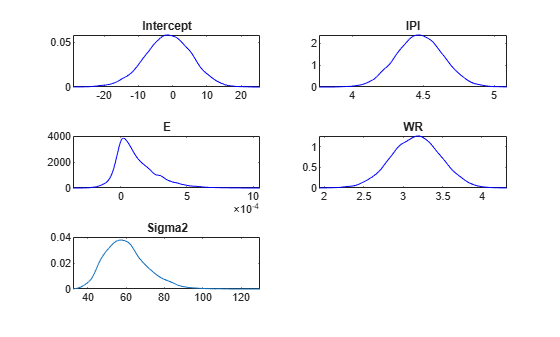Given a shrinkage of 10, the distribution of E is fairly dense around 0. Therefore, E might not be an important predictor.

## Input Arguments

collapse all

Number of predictor variables in the Bayesian multiple linear regression model, specified as a nonnegative integer.

NumPredictors must be the same as the number of columns in your predictor data, which you specify during model estimation or simulation.

When counting the number of predictors in the model, exclude the intercept term specified by Intercept. If you include a column of ones in the predictor data for an intercept term, then count it as a predictor variable and specify 'Intercept',false.

Data Types: double

### Name-Value Arguments

Specify optional comma-separated pairs of Name,Value arguments. Name is the argument name and Value is the corresponding value. Name must appear inside quotes. You can specify several name and value pair arguments in any order as Name1,Value1,...,NameN,ValueN.

Example: 'ModelType','conjugate','Mu',1:3,'V',1000*eye(3),'A',1,'B',0.5 specifies that the prior distribution of Beta given Sigma2 is Gaussian with mean vector 1:3 and covariance matrix Sigma2*1000*eye(3), and the distribution of Sigma2 is inverse gamma with shape 1 and scale 0.5.
Options for All Prior Distributions

collapse all

Joint prior distribution of (β,σ2), specified as the comma-separated pair consisting of 'ModelType' and a value in the following tables.

For a standard Bayesian regression model, choose a value in this table.

ValueDescription
'conjugate'

Normal-inverse-gamma conjugate model

• The prior distributions are

$\begin{array}{l}\beta |{\sigma }^{2}~{N}_{p+1}\left(\mu ,{\sigma }^{2}V\right).\\ {\sigma }^{2}~IG\left(A,B\right).\end{array}$

β and σ2 are dependent.

• The corresponding marginal and conditional posterior distributions have closed forms (see Analytically Tractable Posteriors).

You can adjust corresponding hyperparameters using the Mu, V, A, and B name-value pair arguments.

'semiconjugate'

Normal-inverse-gamma semiconjugate model

• The prior distributions are

$\begin{array}{l}\beta |{\sigma }^{2}~{N}_{p+1}\left(\mu ,V\right).\\ {\sigma }^{2}~IG\left(A,B\right).\end{array}$

β and σ2 are independent.

• The corresponding marginal posterior distribution does not have a closed form, but conditional posterior distributions do (see Analytically Tractable Posteriors).

You can adjust corresponding hyperparameters using the Mu, V, A, and B name-value pair arguments.

'diffuse'

Diffuse prior distributions

• The joint prior pdf is

${f}_{\beta ,{\sigma }^{2}}\left(\beta ,{\sigma }^{2}\right)\propto \frac{1}{{\sigma }^{2}}.$

• The corresponding marginal and conditional posterior distributions have closed forms (see Analytically Tractable Posteriors).

'empirical'

Custom prior distributions

• You must also specify the BetaDraws and Sigma2Draws name-value pair arguments.

• The corresponding marginal and conditional posterior distributions do not have closed forms.

• Empirical models are better suited for updating a posterior distribution based on new data.

'custom'

Custom prior distributions

• You must also specify the LogPDF name-value pair argument.

• The corresponding marginal and conditional posterior distributions do not have closed forms.

For a Bayesian regression model that performs predictor variable selection, choose a value in this table.

ValueDescription
'mixconjugate'

Stochastic search variable selection (SSVS)  conjugate prior distributions

• The data likelihood, prior distribution, and posterior distributions compose a conjugate Gaussian mixture model.

• β and σ2 are dependent random variables.

For more details, see mixconjugateblm.

'mixsemiconjugate'

SSVS  semiconjugate prior distributions

• The data likelihood, prior distribution, and posterior distributions compose a semiconjugate Gaussian mixture model.

• β and σ2 are independent random variables.

For more details, see mixsemiconjugateblm.

'lasso'

Bayesian lasso regression prior distributions 

• Conditioned on σ2, the prior distribution of each regression coefficient is double exponential with a mean of 0 and scale σ/λ, where λ is the lasso shrinkage parameter. As λ increases, the coefficients tend towards 0.

• β and σ2 are dependent random variables. Regression coefficients are independent, a priori.

The prior model type that you choose depends on your assumptions on the joint distribution of the parameters. Your choice can affect posterior estimates and inferences. For more details, see Implement Bayesian Linear Regression.

Example: 'ModelType','conjugate'

Data Types: char

Flag for including a regression model intercept, specified as the comma-separated pair consisting of 'Intercept' and a value in this table.

ValueDescription
falseExclude an intercept from the regression model. Therefore, β is a p-dimensional vector, where p is the value of NumPredictors.
trueInclude an intercept in the regression model. Therefore, β is a (p + 1)-dimensional vector. This specification causes a T-by-1 vector of ones to be prepended to the predictor data during estimation and simulation.

If you include a column of ones in the predictor data for an intercept term, then specify 'Intercept',false.

Example: 'Intercept',false

Predictor variable names for displays, specified as the comma-separated pair consisting of 'VarNames' and a string vector or cell vector of character vectors. VarNames must contain NumPredictors elements. VarNames(j) is the name of the variable in column j of the predictor data set, which you specify during estimation, simulation, or forecasting.

The default is {'Beta(1)','Beta(2)',...,'Beta(p)'}, where p is the value of NumPredictors.

Note

You cannot set the name of the intercept or disturbance variance. In displays, bayeslm gives the intercept the name Intercept and the disturbance variance the name Sigma2. Therefore, you cannot use "Intercept" and "Sigma2" as predictor names.

Example: 'VarNames',["UnemploymentRate"; "CPI"]

Data Types: string | cell | char

Options for Conjugate and Semiconjugate Joint Prior Distribution of β

collapse all

Mean hyperparameter of the Gaussian prior on β, specified as the comma-separated pair consisting of 'Mu' and a numeric vector.

If Mu is a vector, then it must have NumPredictors or NumPredictors + 1 elements.

• For NumPredictors elements, bayeslm sets the prior mean of the NumPredictors predictors only. Predictors correspond to the columns in the predictor data (specified during estimation, simulation, or forecasting). bayeslm ignores the intercept in the model, that is, bayeslm specifies the default prior mean to any intercept.

• For NumPredictors + 1 elements, the first element corresponds to the prior mean of the intercept, and all other elements correspond to the predictors.

Example: 'Mu',[1; 0.08; 2]

Data Types: double

Conditional covariance matrix hyperparameter of the Gaussian prior on β, specified as the comma-separated pair consisting of 'V' and a c-by-c symmetric, positive definite matrix. c can be NumPredictors or NumPredictors + 1.

• If c is NumPredictors, then bayeslm sets the prior covariance matrix to

$\left[\begin{array}{cccc}1e5& 0& \cdots & 0\\ 0& & & \\ ⋮& & V& \\ 0& & & \end{array}\right].$

bayeslm attributes the default prior covariances to the intercept, and attributes V to the coefficients of the predictor variables in the data. Rows and columns of V correspond to columns (variables) in the predictor data.

• If c is NumPredictors + 1, then bayeslm sets the entire prior covariance to V. The first row and column correspond to the intercept. All other rows and columns correspond to the columns in the predictor data.

The default value is a flat prior. For an adaptive prior, specify diag(Inf(Intercept + NumPredictors,1)). Adaptive priors indicate zero precision in order for the prior distribution to have as little influence as possible on the posterior distribution.

For 'ModelType',conjugate, V is the prior covariance of β up to a factor of σ2.

Example: 'V',diag(Inf(3,1))

Data Types: double

Options for Bayesian Lasso Regression

collapse all

Lasso regularization parameter for all regression coefficients, specified as the comma-separated pair consisting of 'Lambda' and a positive numeric scalar or (Intercept + NumPredictors)-by-1 positive numeric vector. Larger values of Lambda cause corresponding coefficients to shrink closer to zero.

Suppose X is a T-by-NumPredictors matrix of predictor data, which you specify during estimation, simulation, or forecasting.

• If Lambda is a vector and Intercept is true, Lambda(1) is the shrinkage for the intercept, Lambda(2) is the shrinkage for the coefficient of the first predictor X(:,1), Lambda(3) is the shrinkage for the coefficient of the second predictor X(:,2),…, and Lambda(NumPredictors + 1) is the shrinkage for the coefficient of the last predictor X(:,NumPredictors).

• If Lambda is a vector and Intercept is false, Lambda(1) is the shrinkage for the coefficient of the first predictor X(:,1),…, and Lambda(NumPredictors) is the shrinkage for the coefficient of the last predictor X(:,NumPredictors).

• If you supply the scalar s for Lambda, then all coefficients of the predictors in X have a shrinkage of s.

• If Intercept is true, the intercept has a shrinkage of 0.01, and lassoblm stores [0.01; s*ones(NumPredictors,1)] in Lambda.

• Otherwise, lassoblm stores s*ones(NumPredictors,1) in Lambda.

Example: 'Lambda',6

Data Types: double

Options for Prior Distribution of β and γ for SSVS Predictor Variable Selection

collapse all

Component-wise mean hyperparameter of the Gaussian mixture prior on β, specified as the comma-separated pair consisting of 'Mu' and an (Intercept + NumPredictors)-by-2 numeric matrix. The first column contains the prior means for component 1 (the variable-inclusion regime, that is, γ = 1). The second column contains the prior means for component 2 (the variable-exclusion regime, that is, γ = 0).

• If Intercept is false, then Mu has NumPredictors rows. bayeslm sets the prior mean of the NumPredictors coefficients corresponding to the columns in the predictor data set, which you specify during estimation, simulation, or forecasting.

• Otherwise, Mu has NumPredictors + 1 elements. The first element corresponds to the prior means of the intercept, and all other elements correspond to the predictor variables.

Tip

To perform SSVS, use the default value of Mu.

Data Types: double

Component-wise variance factor or variance hyperparameter of the Gaussian mixture prior on β, specified as the comma-separated pair consisting of 'V' and an (Intercept + NumPredictors)-by-2 positive numeric matrix. The first column contains the prior variance factors for component 1 (the variable-inclusion regime, that is, γ = 1). The second column contains the prior variance factors for component 2 (the variable-exclusion regime, that is, γ = 0). For conjugate models ('ModelType','mixconjugate'), V contains variance factors, and for semiconjugate models ('ModelType','mixsemiconjugate'), V contains variances.

• If Intercept is false, then V has NumPredictors rows. bayeslm sets the prior variance factor of the NumPredictors coefficients corresponding to the columns in the predictor data set, which you specify during estimation, simulation, or forecasting.

• Otherwise, V has NumPredictors + 1 elements. The first element corresponds to the prior variance factor of the intercept, and all other elements correspond to the predictor variables.

Tip

• To perform SSVS, specify a larger variance factor for regime 1 than for regime 2. That is, for all j, specify V(j,1) > V(j,2).

• For details on what value to specify for V, see .

Data Types: double

Prior probability distribution for the variable inclusion and exclusion regimes, specified as the comma-separated pair consisting of 'Probability' and an (Intercept + NumPredictors)-by-1 numeric vector of values in [0,1], or a function handle in the form @fcnName, where fcnName is the function name. Probability represents the prior probability distribution of γ = {γ1,…,γK}, where:

• K = Intercept + NumPredictors, which is the number of coefficients in the regression model.

• γk ∈ {0,1} for k = 1,…,K. Therefore, the sample space has a cardinality of 2K.

• γk = 1 indicates variable VarNames(k) is included in the model, and γk = 0 indicates that the variable is excluded from the model.

If Probability is a numeric vector:

• Rows correspond to the variable names in VarNames. For models containing an intercept, the prior probability for intercept inclusion is Probability(1).

• For k = 1,…,K, the prior probability for excluding variable k is 1 – Probability(k).

• Prior probabilities of the variable-inclusion regime, among all variables and the intercept, are independent.

If Probability is a function handle, then it represents a custom prior distribution of the variable-inclusion regime probabilities. The corresponding function must have this declaration statement (the argument and function names can vary):

logprob = regimeprior(varinc)

• logprob is a numeric scalar representing the log of the prior distribution. You can write the prior distribution up to a proportionality constant.

• varinc is a K-by-1 logical vector. Elements correspond to the variable names in VarNames and indicate the regime in which the corresponding variable exists. varinc(k) = true indicates VarName(k) is included in the model, and varinc(k) = false indicates it is excluded from the model.

You can include more input arguments, but they must be known when you call bayeslm.

For details on what value to specify for Probability, see .

Data Types: double | function_handle

Prior correlation matrix of β for both components in the mixture model, specified as the comma-separated pair consisting of 'Correlation' and

an (Intercept + NumPredictors)-by-(Intercept + NumPredictors) numeric, positive definite matrix. Consequently, the prior covariance matrix for component j in the mixture model is:

• For conjugate ('ModelType','mixconjugate'), sigma2*diag(sqrt(V(:,j)))*Correlation*diag(sqrt(V(:,j)))

• For semiconjugate ('ModelType','mixsemiconjugate'), diag(sqrt(V(:,j)))*Correlation*diag(sqrt(V(:,j)))

where sigma2 is σ2 and V is the matrix of coefficient variance factors or variances.

Rows and columns correspond to the variable names in VarNames.

By default, regression coefficients are uncorrelated, conditional on the regime.

Note

You can supply any appropriately sized numeric matrix. However, if your specification is not positive definite, bayeslm issues a warning and replaces your specification with CorrelationPD, where:

CorrelationPD = 0.5*(Correlation + Correlation.');

Tip

For details on what value to specify for Correlation, see .

Data Types: double

Options for Prior Distribution of σ2

collapse all

Shape hyperparameter of the inverse gamma prior on σ2, specified as the comma-separated pair consisting of 'A' and a numeric scalar.

A must be at least –(Intercept + NumPredictors)/2.

With B held fixed, the inverse gamma distribution becomes taller and more concentrated as A increases. This characteristic weighs the prior model of σ2 more heavily than the likelihood during posterior estimation.

For the functional form of the inverse gamma distribution, see Analytically Tractable Posteriors.

This option does not apply to empirical or custom prior distributions.

Example: 'A',0.1

Data Types: double

Scale hyperparameter of the inverse gamma prior on σ2, specified as the comma-separated pair consisting of 'B' and a positive scalar or Inf.

With A held fixed, the inverse gamma distribution becomes taller and more concentrated as B increases. This characteristic weighs the prior model of σ2 more heavily than the likelihood during posterior estimation.

This option does not apply to empirical or custom prior distributions.

Example: 'B',5

Data Types: double

Required Options for Empirical Joint Prior Distributions

collapse all

Random sample from the prior distribution of β, specified as the comma-separated pair consisting of 'BetaDraws' and an (Intercept + NumPredictors)-by-NumDraws numeric matrix. Rows correspond to regression coefficients: the first row corresponds to the intercept, and the subsequent rows correspond to columns in the predictor data. Columns correspond to successive draws from the prior distribution.

BetaDraws and Sigma2Draws must have the same number of columns.

For best results, draw a large number of samples.

Data Types: double

Random sample from the prior distribution of σ2, specified as the comma-separated pair consisting of 'Sigma2Draws' and a 1-by-NumDraws numeric row vector. Columns correspond to successive draws from the prior distribution.

BetaDraws and Sigma2Draws must have the same number of columns.

For best results, draw a large number of samples.

Data Types: double

Required Options for Custom Prior Distributions

collapse all

Log of the joint probability density function of (β,σ2), specified as the comma-separated pair consisting of 'LogPDF' and a function handle.

Suppose logprior is the name of the MATLAB® function defining the joint prior distribution of (β,σ2). Then, logprior must have this form.

function [logpdf,glpdf] = logprior(params) ... end
where:

• logpdf is a numeric scalar representing the log of the joint probability density of (β,σ2).

• glpdf is an (Intercept + NumPredictors + 1)-by-1 numeric vector representing the gradient of logpdf. Elements correspond to the elements of params.

glpdf is an optional output argument, and only the Hamiltonian Monte Carlo sampler (see hmcSampler) applies it. If you know the analytical partial derivative with respect to some parameters, but not others, then set the elements of glpdf corresponding to the unknown partial derivatives to NaN. MATLAB computes the numerical gradient for missing partial derivatives, which is convenient, but slows sampling.

• params is an (Intercept + NumPredictors + 1)-by-1 numeric vector. The first Intercept + NumPredictors elements must correspond to values of β, and the last element must correspond to the value of σ2. The first element of β is the intercept, if one exists. All other elements correspond to predictor variables in the predictor data, which you specify during estimation, simulation, or forecasting.

Example: 'LogPDF',@logprior

## Output Arguments

collapse all

Bayesian linear regression model storing prior model assumptions, returned as an object in this table.

Value of ModelTypeReturned Bayesian Linear Regression Model Object
'conjugate'conjugateblm
'semiconjugate'semiconjugateblm
'diffuse'diffuseblm
'empirical'empiricalblm
'custom'customblm
'mixconjugate'mixconjugateblm
'mixsemiconjugate'mixsemiconjugateblm
'lasso'lassoblm

PriorMdl specifies the joint prior distribution and characteristics of the linear regression model only. The model object is a template intended for further use. To incorporate data into the model for posterior distribution analysis, pass the model object and data to the appropriate object function, for example, estimate or simulate.

collapse all

### Bayesian Linear Regression Model

A Bayesian linear regression model treats the parameters β and σ2 in the multiple linear regression (MLR) model yt = xtβ + εt as random variables.

For times t = 1,...,T:

• yt is the observed response.

• xt is a 1-by-(p + 1) row vector of observed values of p predictors. To accommodate a model intercept, x1t = 1 for all t.

• β is a (p + 1)-by-1 column vector of regression coefficients corresponding to the variables that compose the columns of xt.

• εt is the random disturbance with a mean of zero and Cov(ε) = σ2IT×T, while ε is a T-by-1 vector containing all disturbances. These assumptions imply that the data likelihood is

$\ell \left(\beta ,{\sigma }^{2}|y,x\right)=\prod _{t=1}^{T}\varphi \left({y}_{t};{x}_{t}\beta ,{\sigma }^{2}\right).$

ϕ(yt;xtβ,σ2) is the Gaussian probability density with mean xtβ and variance σ2 evaluated at yt;.

Before considering the data, you impose a joint prior distribution assumption on (β,σ2). In a Bayesian analysis, you update the distribution of the parameters by using information about the parameters obtained from the likelihood of the data. The result is the joint posterior distribution of (β,σ2) or the conditional posterior distributions of the parameters.

 George, E. I., and R. E. McCulloch. "Variable Selection Via Gibbs Sampling." Journal of the American Statistical Association. Vol. 88, No. 423, 1993, pp. 881–889.

 Koop, G., D. J. Poirier, and J. L. Tobias. Bayesian Econometric Methods. New York, NY: Cambridge University Press, 2007.

 Park, T., and G. Casella. "The Bayesian Lasso." Journal of the American Statistical Association. Vol. 103, No. 482, 2008, pp. 681–686.